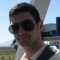Home » Core Java » lang » Java equals method – .equals Java ExampleAldo is a student of Computer Engineering and a programming addict. He spares his free time coding, whether mobile, web, or desktop programming. He is also one of the co-founders of Things Lab.

# Java equals method – .equals Java Example

In this example we will discuss about the Java equals method: .equals Java method. This is one of the methods that all objects have, since it is defined in the `Object` class, which is the base for all Java classes.

This method’s functionality is to check if the object invoking this method is equal to another object passed as an argument. It should return `true` if the objects are equal, otherwise it should return `false`.

It is generally necessary to override the `hashCode()` method whenever the `equals()` method is overridden, so as to maintain the general contract for the `hashCode()` method, which states that equal objects must have equal hash codes.

The `equals()` method for the `Object` class implements the most discriminating possible equivalence relation on objects; that is, for any non-null reference values `x` and `y`, this method returns true if and only if `x` and `y` refer to the same object (`x == y` has the value `true`).

## 1. Example of .equals Java method

In this example, I will show that for two references `x` and `y`, when `x == y` is `true`, also `x.equals(y)` is `true`, but the opposite is not always true.
Create a class called `BasicEqualsExample` with the following source code:

 0102030405060708091011121314151617 `package` `com.javacodegeeks.example;` `public` `class` `BasicEqualsExample {` `    ``public` `static` `void` `main(String[] args) {``        ``String s1 = ``new` `String(``"John"``);``        ``String s2 = ``new` `String(``"John"``);``        ``String s3 = s1; ``//references of the same object``        ` `        ``System.out.println(``"s1 == s2: "``+(s1 == s2));``        ``System.out.println(``"s1.equals(s2): "``+(s1.equals(s2)));``        ``System.out.println(``"s1 == s3: "``+(s1 == s3));``        ``System.out.println(``"s1.equals(s3): "``+(s1.equals(s3)));` `    ``}` `}`

On this example, I created 3 new instances of the `String` class. Two of them are references to the same object, (`s1` and `s3`), while two others have the same content, but are different objects (`s1` and `s2`).
As you can see, the `==` operator returns true only when the objects refer to the same address in the memory, without checking the content of the memory. On the other hand, the `equals()` method doesn’t care about the memory address; it checks the values of the objects, not their references.
The output, is this:

 1234 `s1 == s2: ``false``s1.equals(s2): ``true``s1 == s3: ``true``s1.equals(s3): ``true`

## 2. Implementing the equals() method

To show a basic implementation of the `equals()` method, I will firstly create a new class, called `Vehicle`, with the following source code:

 010203040506070809101112131415161718192021222324 `package` `com.javacodegeeks.example.impl;` `public` `class` `Vehicle {``    ``private` `String model;``    ``private` `int` `yearOfProduction;``    ``private` `String manufacturer;``    ` `    ``public` `Vehicle() {}``    ` `    ``public` `Vehicle(String model, ``int` `yearOfProduction, String manufacturer) {``        ``this``.model = model;``        ``this``.yearOfProduction = yearOfProduction;``        ``this``.manufacturer = manufacturer;``    ``}``    ` `    ``public` `boolean` `equals(Vehicle v) {``        ``if` `(v == ``null``) ``return` `false``;``        ` `        ``return` `((``this``.model).equals(v.model)``                ``&& (``this``.yearOfProduction == v.yearOfProduction)``                ``&& (``this``.manufacturer).equals(v.manufacturer));``    ``}` `}`

You can see the implementation of the `equals()` method there, but to see it in action, I created another class, called `Main`, with this source code:

 0102030405060708091011121314151617181920 `package` `com.javacodegeeks.example.impl;` `public` `class` `Main {` `    ``public` `static` `void` `main(String[] args) {``        ``Vehicle myCar = ``new` `Vehicle(``"Focus"``,``2002``,``"Ford"``);``        ``Vehicle minivan = ``new` `Vehicle (``"Odyssey"``,``2014``,``"Honda"``);``        ``Vehicle focus = ``new` `Vehicle(``"Focus"``,``2002``,``"Ford"``);``        ` `        ``if` `(myCar.equals(minivan)) {``            ``System.out.println(``"This isn't supposed to print!"``);``        ``}``        ` `        ``if` `(myCar.equals(focus)) {``            ``System.out.println(``"The equals method is implemented OK"``);``        ``}` `    ``}` `}`

As you expected, the output will be this:

 1 `The equals method is implemented OK`

## 3. More about the .equals Java method

The equals method implements an equivalence relation on non-null object references:

• It is reflexive: for any non-null reference value x, `x.equals(x)` should return true.
• It is symmetric: for any non-null reference values x and y, `x.equals(y)` should return `true` if and only if `y.equals(x)` returns `true`.
• It is transitive: for any non-null reference values x, y, and z, if `x.equals(y)` returns `true` and `y.equals(z)` returns `true`, then `x.equals(z)` should return `true`.
• It is consistent: for any non-null reference values x and y, multiple invocations of `x.equals(y)` consistently return true or consistently return false, provided no information used in equals comparisons on the objects is modified.
• For any non-null reference value x, `x.equals(null)` should return false.

Now, I will test my `equals()` implementation against all of the above:

 0102030405060708091011121314151617181920212223242526272829303132333435363738394041424344454647 `package` `com.javacodegeeks.example.impl;` `public` `class` `VehicleTest {``    ` `    ``public` `static` `void` `main(String[] args) {``        ``Vehicle x = ``new` `Vehicle(``"Focus"``,``2002``,``"Ford"``);``        ``Vehicle y = ``new` `Vehicle(``"Focus"``,``2002``,``"Ford"``);``        ``Vehicle z = ``new` `Vehicle(``"Focus"``,``2002``,``"Ford"``);``        ` `        ``int` `correctCases = ``0``;` `        ``//reflexiveness test``        ``if` `(x.equals(x)) {``            ``System.out.println(``"It is reflexive!"``);``            ``correctCases++;``        ``} ``else` `{``            ``System.out.println(``"It is not reflexive"``);``        ``}``        ` `        ``//symmetry test``        ``if` `(x.equals(y) && y.equals(x)) {``            ``System.out.println(``"It is symmetric!"``);``            ``correctCases++;``        ``} ``else` `{``            ``System.out.println(``"It is not symmetric"``);``        ``}``        ` `        ``//transitiveness test``        ``boolean` `cause1 = x.equals(y) && y.equals(z);``        ``if` `(cause1 == x.equals(z)) {``            ``System.out.println(``"It is transitive!"``);``            ``correctCases++;``        ``} ``else` `{``            ``System.out.println(``"It is not transitive"``);``        ``}``        ` `        ``if` `(x.equals(``null``) == ``false``) {``            ``System.out.println(``"Nothing equals null"``);``            ``correctCases++;``        ``} ``else` `{``            ``System.out.println(``"An object equals null?!"``);``        ``}``        ` `        ``System.out.println(correctCases+``"/4 correct cases"``);``    ``}` `}`

Fortunately, my method is correctly implemented, and the output is this:

 12345 `It is reflexive!``It is symmetric!``It is transitive!``Nothing equals null``4``/4` `correct cases`

I didn’t test for the consistency of the method, because it is actually obvious: if the properties of `x` and `y` objects don’t change, the `equals()` method will return the same value.

You can download the full source code of this example here: Java equals method – .equals Java Example

Last updated on Feb. 17th, 2020

# Do you want to know how to develop your skillset to become a Java Rockstar?

## Subscribe to our newsletter to start Rocking right now!

### and many more ....0 Followers

Most reacted commentRecent comment authors